Kang: Word Similarity Calculation by Using the Edit Distance Metrics with Consonant Normalization

### Abstract

Edit distance metrics are widely used for many applications such as string comparison and spelling error corrections. Hamming distance is a metric for two equal length strings and Damerau-Levenshtein distance is a well-known metrics for making spelling corrections through string-to-string comparison. Previous distance metrics seems to be appropriate for alphabetic languages like English and European languages. However, the conventional edit distance criterion is not the best method for agglutinative languages like Korean. The reason is that two or more letter units make a Korean character, which is called as a syllable. This mechanism of syllable-based word construction in the Korean language causes an edit distance calculation to be inefficient. As such, we have explored a new edit distance method by using consonant normalization and the normalization factor.

### 1. Introduction

While we are using digital devices like smart phones, many users often make spelling or phonetic errors and they tend to be more forgiving if someone else makes the errors. It is especially common for teenagers write a word as it sounds. In this error-prone environment, we need a method for making an automatic correction of the misspelled words. When we are able to find and correct these errors, the edit distance metric can be applied. In this paper, we tried to apply a modified edit distance metric that could meet our goal. Edit distance is a theoretically important issue when we need to compare the phonetic similarity between two words and to correct typos in a text. It is widely used for the automatic correction of word-spacing errors, spelling errors, and classifying the phonemes into groups. Edit distance is the unit operation cost that is required to transform one string to another string in which those two strings become the same string. There are four unit operations: insertion, deletion, replacement, and transposition. The insertions and deletions have equal costs and replacements have twice the cost of an insertion [1,2].
Edit distance is widely used for string-to-string comparison in alphabetic languages, such as English and European languages. However, the conventional edit distance metric is not the best method for agglutinative languages like Korean [3,4]. We investigated the reason for this and discovered that a word construction mechanism should be considered for using the edit distance calculation. In the Korean language, two or more letter units make a Korean character, which is called a syllable. An open syllable consists of a consonant followed by a vowel, and a close syllable consists of three letters—consonant + vowel + consonant. Furthermore, when we write a complex consonant or a complex vowel, two keystrokes are required. That is, a syllable is completed in a computer environment by two to five keystrokes from an input device like a keyboard or mouse. This mechanism of syllable construction in the Korean language causes the edit distance calculation to be inefficient. As such, we explored a new edit distance method by using consonant normalization and the normalization factor.
The input mechanism of complex consonants and complex vowels requires two keystrokes on a keyboard, but they are one of the components in a Korean syllable. This is what makes it difficult to set a specific guideline for calculating the edit distance. Suppose that there are two complex vowels “” (wa) and “” (wo). The vowel “” (wa) is a combination of “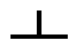”(o) and “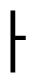”(a). Also, “”(wa) is a combination of “” and “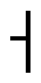.” When “” is substituted by “.” There are two candidates for calculating an edit distance. The edit distance would be 1.0 because only one of the letters in a syllable is being substituted. However, it may be 0.5 because half of the complex vowel is different from the keystroke point of view, “” and “.” This kind of word construction characteristic can be considered for a word distance metric.

### 2. Related Work

Edit distance is used to calculate the distance between two words by insertion, deletion, and substitution. For example, the only difference in the English words “best” and “beast” is the letter “a.” We can easily make a mistake of writing “beast” instead of “best.” A word “best” is written as “beast” by inserting “a” when we press the “s” key because “a” is the closest key to “s.” The distance between “best” and “beast” is calculated as shown in Fig. 1.
There are several distance measures: Hamming distance, Levenshtein distance, Damerau-Levenshtein distance, and Jaro-Winkler distance. Hamming distance is a metric between two strings of equal length . It is used in information theory to count the number of substitutions while transferring a text string. Edit distance is often used to refer to Levenshtein distance, which is a mathematical measure for calculating the difference between two strings . Levenshtein assumes that the transmission of binary information usually considers a channel model in which 0→1 and 1→0 substitutions are admitted. Levenshtein’s distance metric is recursively defined as shown in Eq. (1), in which distance measures are insertion, deletion, and substitution.
Damerau focused his research on human spelling errors and added a transposition operation between two adjacent characters . That is, Damerau-Levenshtein distance is an extension of the Levenshtein distance by adding a new operation of transposition. Jaro-Winkler distance has been designed and is best suited for short strings, such as the names of people . The Jaro-Winkler score is 0 with no similarity and 1 with an exact match. Eq. (2) describe the Jaro distance dj of two given strings s1 and s2, where m is the number of matching characters and t is half the number of transpositions.
##### (1)
dista,b(i,j)={0,i=j=0i,j=0andi>0j,i=0andj>0min{dista,b(i-1,j)+1dista,b(i,j-1)+1dista,b(i-1,j-1)+1(aibj),otherwise
##### (2)
dj={0ifm=012(ms1+ms2+m-tm)otherwise

### 3. Edit Distance for Korean Words

A Korean word is a sequence of syllables, which is called known as a Korean character. A Korean syllable is a combination of consonants (C) and vowels (V) in which only the C1VC2 format is allowed. In this syllable construct, the final consonant C2 is not mandatory. As such, there are two categories of syllable types, which are C1V and C1VC2. The syllable form of C1V is called an “open syllable” and the C1VC2 form is called a “closed syllable.” Basically, there are 14 consonants, but some of the two consonants are combined to make a complex consonant. Only 19 consonants are used for C1 and 27 consonants are used for C2. There are 21 vowels in Korean, which are 10 base vowels and 11 complex vowels. The possible combinations of C1V and C1VC2 are 19×21×28. That is, a total of 11,172 syllables are used in the Korean language.
A Korean character can be considered to be very similar to Chinese character in that it consists of one or more basic letter units. However, the internal structure of a Korean character is altogether different from a Chinese character. Because the Korean character system is a well-formed and loosely combined letter system, it can be separated into letters of consonant and vowels. On the opposite hand, Chinese characters cannot be divided into letters. Cha  analyzed the distance measures and proposed the distance learning method. The general edit distance metric has been devised and applied for a simple, linear, and one-dimensional string like English words [10,11]. When we try to apply this edit distance metric to the two-dimensional Korean word system, three methods are possible according to the operand types for basic operations.

### 3.1 Letter-Based Edit Distance

The letter-based method applies the conventional Levenshtein distance to a letter string. A word is considered to be a sequence of consonants or vowels with no consideration of syllable construction. Fig. 2 shows an example of letter-based distance. The distance of “”(baseball play) and “”(volleyball play) is 2 because two letters need to be changed to match the words.
This method ignores the syllable boundary that the distance calculation for misspelled or transposition errors across the syllable boundaries are the same as the edit distance calculation inside a syllable. The words “” and “” are frequent typos in Korean sentences that are generated by transposition errors when a person types “”. The word “” is composed as a sequence of the letters “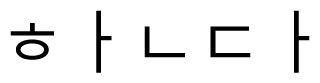” and the transposition of “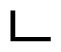” and “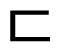” will create the word “.” In the same way, the transposition of “” and “” creates the word ‘’.
The letter-based metric easily computes transposition errors that cannot be handled in a syllable-based metric, but it does not consider the syllable characteristics of the Korean language where each word is a sequence of well-formed syllables of CV or C1VC2. The syllable-based system naturally puts a limitation on consonant and vowel combinations. Therefore, most typos are affected by the syllable formation rules. That is why the edit distance metric needs to reflect these kinds of syllable-structured characteristics.

### 3.2 Syllable-Based Edit Distance

A Korean word is defined as a sequence of syllables. The syllable-based edit distance is used to calculate the number of different syllables in a string. This metric is adequate for long strings, like text-to-text similarity, but it cannot be used for short strings. This is because all of the distance scores are 0 or 1, regardless of the similarity of syllables. The difference between the syllables “” and “” is the final consonant “ “(null) and “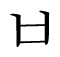” which is one third of the syllable. In other example using “” and “,” these two syllables are entirely different for all of the letters that constitute a syllable. However, syllable-based distances d(“,” “”) and d(“,” “”) have the same score. Furthermore, the syllable-based method has a critical problem when typos occur in a syllable boundary. The operations in the syllable-based metric are insertion, deletion, and substitution.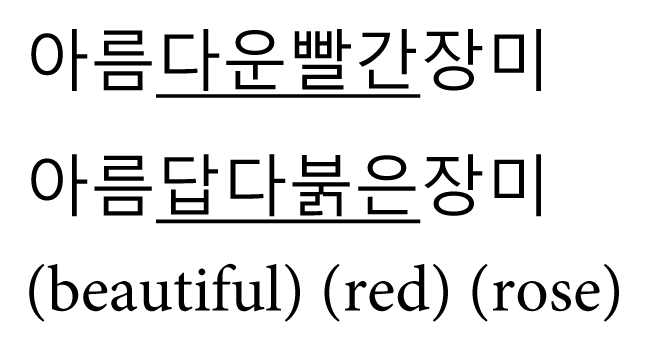The difference between the letter-based metric and syllable-based metric is the edit distance calculation unit. Fig. 3 is an example of the syllable-based distance for the same example in Fig. 2.

### 3.3 Hybrid Edit Distance

Roh et al.  points out the inefficiencies of the syllable-based and letter-based methods. The syllable-based metric has a drawback in which only one letter difference and an entire syllable difference are treated as the same distance, as illustrated in the example of dsyl() = dsyl() = 1. In this example, dsyl(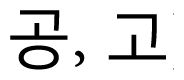) is the same distance as dsyl(), even though there are two matching letters in “” and “,” where there is no matching letter in “” and “.” Fig. 4 is an example of the hybrid edit distance for the same words that are shown in Figs. 2 and 3.
The letter-based metric is not able to provide an accurate distance measurement when a whole syllable is missing. If a target syllable consists of two letters then the edit distance is 2, but the distance is 3 for three-lettered syllables. Roh et al.  proposed a hybrid method of a syllable and letter-based metric. He defined β–distance for the syllable-based metric and α-distance for the letter-based metric. If there are one or two matching letters, then α-distance is applied, and β–distance is used for the entire syllable difference. In the letter-based metric, three letter-distance 3α is the maximum in one syllable that 3α is considered to be one β–distance.

### 4. Phoneme-Based Edit Distance

The hybrid edit distance has been devised for the syllable structure of the Korean language. This method divides the distance metric into syllable distance and letter distance. By doing so, edit distance has been specified in detail. However, this hybrid method did not solve the syllable-boundary changes. In this paper, we propose a new method that considers the phonetic change rules of the Korean language. It is common for the normalization technique to be widely used for the unfair comparison of two objectives [12,13].

### 4.1 Phoneme Normalization

Our new approach for a Korean edit distance calculation considers phonetic pronunciation. In order to preprocess input strings, a final consonant can be normalized to a phoneme representative. Phoneme normalization is based on the Korean letter system’s final consonants and pronunciation rules. In this approach, nine phonetic transformation rules by Lee (14) are sequentially applied. After that, the letter-based distance metric is applied to the modified input strings. Table 1 shows phonetically equal consonants and their corresponding representatives.

### 4.2 Edit Distance Normalization

We have introduced edit distance normalization for two reasons. First, the word-length independent metric will obtain a better result than the word-length dependent metric. Second, non-similar words are easily filtered out when there are lots of word pairs for comparison candidates.
Fig. 5 shows two examples of edit distance calculations. The meaning of edit distance 1 in these two cases is not the same. It is common that the edit distance of 1 or 2 between two short words shows that these two words are different words, but the possibility of two words meaning the same increases as the word-length increases. In Fig. 1, the meaning of “” (national language) and “” (phrase) are different, where “” (“tree branch” in the old spelling standard) and “” (“tree branch” in the new spelling standard) have the same meaning. In order to increase the discrimination power of the edit distance metric, word-length normalization has been introduced. In Eq. (3), α is an edit distance and |wi| is a word length.
##### (3)
N=α/max(w1,w2)wherewi=3×syl_length(wi)
Word length |wi| is the syllable length of the word multiplied by 3 in which the length of a syllable is defined as 3. The range of N is between 0 and 1. Zero means that two word strings are equal and 1 means that they are altogether different. For the example word pairs in Fig. 1, the distance normalization score of <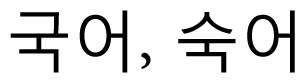> is 0.16(=1/6) and that of <> is 0.08(=1/12). These distance values mean that a long word-length pair is more similar than a short word-length pair.

### 5. Experiments and Results

We performed an experiment for confusing word pairs in terms of finding out whether our metric makes a correct decision of if it is a confusing word pair or not. Test data was collected from the real field where people commonly misuse the words. Experiments on normalization effects between two similar word pairs were conducted. The amount of test data was 699 confusing word pairs that were created by the Ministry of Culture and Tourism . Non-similar word pairs were automatically constructed by all of the other words, except the ones in the similar word pairs. That is, non-similar word pairs are 698 for each word. Fig. 6 shows the normalization effect. The x-axis is the threshold value for the similarity decision and the y-axis is the percentage of word pairs according to the threshold value. If we make a decision that a normalization score is less than 0.4, then 93.56% of word pairs are determined to be the synonym word.
Fig. 7 shows experimental results for 243,951 non-similar word pairs. For threshold value 0.4, 0.08% of word pairs were determined to be similar and 0.03% for the threshold value of the 0.3. x-axis and y-axis are the same as shown in Fig. 6. The only difference is the ratio of similar or non-similar words. This experiment is for non-similar words where the lowest value is the best result. The best result was that 99.97% of non-similar words were classified as non-similar for the normalization value of 0.3. Figs. 6 and 7 shows the results of our experiments for the similar words and non-similar words, respectively. In the real field, similar and non-similar words are mixed. Therefore, we combined those two separate results and they are shown in Fig. 8. The threshold value for a similar or non-similar word pair is controversy that we should induce the best threshold value. Fig. 8 shows the result of comparing the accuracy and the threshold value, where 0.5 got the best result. This experiment was performed for 243,951 non-similar word pairs and 242,732 word pairs were correctly determined.
We performed an experiment on Korean word pairs and the results are shown in Tables 2 and 3. The threshold value of the proposed method was set to 0.5. The methods in Tables 2 and 3 are as follows: LetED is a baseline method that is commonly used as a distance metric and SylED is a syllable-based method where words are considered to be a sequence of syllables. SylED is based on the syllable-structure of the Korean language, but it doesn’t take the CVC letter units into consideration.
LetED is the basic distance method, but it ignores the syllable boundary. HybED is a combination of LetED and SylED that reflects the characteristics of the syllable-based writing system. PhoED is an extension of HybED that adds phoneme pronunciation rules. NphoED adds one more feature of final consonant normalization. Table 2 shows that the phoneme-based and normalized phoneme-based metrics obtained a better result than the other methods. For the distances 2 and 5, the phoneme-based metric with normalization is especially better than the other metrics.
Table 2 shows that the ratio of distance 0 for a similar word set in the NphoED method is 22.3%. It is 8.1% better than PhoED and it appears to be that NphoED is the best method. The NphoED method showed that word similarity accuracy is a bit better than the other methods for the edit distances of 1 and 2. This metric achieved the best performance with 91.6% in the edit distance of 3 and 98.6% in the edit distance of 6. Table 3 shows the experiment that we conducted for non-similar word pairs. In this experiment, the lowest value is the best one and the proposed metric is acceptable.

### 6. Conclusion

Although edit distance metric is a well-known method for string-to-string comparison and is good for alphabetic languages, the method is not appropriate for syllable-based languages like Korean. This is because a syllable-based language has a sub-structure inside a word string. We have proposed a new method of a word similarity calculation method for syllable-structured languages. This metric was devised to improve the performance of the syllable-based and letter-based metrics, especially for the word similarity calculation. The accuracy of the word similarity calculation was improved through the phonetic transformation by pronunciation rules and word-length normalization. We performed an experiment for confusing word pairs in terms of whether our metric makes a correct decision for it being a confusing word pair or not. Test data was collected from the real field where people commonly misspelled words. Our experimental results show that the phoneme-based normalization method is better than other methods for both similar word pairs and non-similar word pairs.

### Biography

He received his B.S. degree in Computer Science from Seoul National University in 1986, and M.S. and Ph.D. degrees in Computer Science from the same University, in 1988 and 1993, respectively. He worked for Hansung University as an associate professor from 1994 to 2001. Currently, he is working for Kookmin University as a full professor. His research interests include natural language processing, information retrieval, text mining, big data processing, and machine learning.### References

1. RA. Wagner, and MJ. Fischer, "The string-to-string correction problem," Journal of the ACM, vol. 21, no. 1, pp. 168-173, 1974.2. G. Navarro, "A guided tour to approximate string matching," ACM Computing Surveys, vol. 33, no. 1, pp. 31-88, 2001.3. K. Roh, JW. Kim, E. Kim, K. Park, and HG. Cho, "Edit distance problem for the Korean alphabet," Journal of Korean Institute of Information Scientists and Engineers: Systems and Theory, vol. 37, no. 2, pp. 103-109, 2010.

4. B. Bae, SS. Kang, and BY. Hwang, "Edit distance calculation by phonetic rules and word-length normalization," in Proceedings of the European Computing Conference (ECC’12), Prague, Czech Republic, 2012, pp. 315-319.

5. RW. Hamming, "Error detecting and error correcting codes," Bell System Technical Journal, vol. 29, no. 2, pp. 147-160, 1950.6. VI. Levenshtein, "Binary codes capable of correcting deletions, insertions and reversals," Soviet Physics-Doklady, vol. 10, no. 8, pp. 707-710, 1966.

7. FJ. Damerau, "A technique for computer detection and correction of spelling errors," Communications of the ACM, vol. 7, no. 3, pp. 171-176, 1964.8. WE. Winkler, "String comparator metrics and enhanced decision rules in the Fellegi-Sunter model of record linkage," Proceedings of the Survey Research Methods Section, 1990;pp. 354-359.

9. SH. Cha, "Taxonomy of nominal type histogram distance measures," in Proceedings of the American Conference on Applied Mathematics, Cambridge, MA, 2008, pp. 325-330.

10. D. Gusfield, in Algorithms on Strings, Trees, and Sequences: Computer Science and Computational Biology, New York, NY: Cambridge University Press, 1997.

11. K. Kukich, "Techniques for automatically correcting words in text," ACM Computing Surveys, vol. 24, no. 4, pp. 377-439, 1992.12. L. Ahmedi, and E. Jajaga, "A database normalization tool using Semantic Web technologies," International Journal of Systems Applications, Engineering & Development, vol. 5, no. 4, pp. 502-517, 2011.

13. AN. Khan, LM. Sheikh, and S. Sarfraz, "Psyche mining with PsycheTagger: a computational linguistics approach to text mining," International Journal of Computers and Communications, vol. 6, no. 2, pp. 119-127, 2012.

14. H. Lee, "A study on the efficient education of pronunciation in Korean phonetic transformation rules,"MS thesis, Dong-A University; Busan, Korea: 2008.

15. S. Chang, S. Kim, and S. Chung, in This Slip of the Tongue that Slip of the Pen, Seoul, Korea: Ministry of Culture and Tourism, 2000.

##### Fig. 1
Example of an edit distance in English words.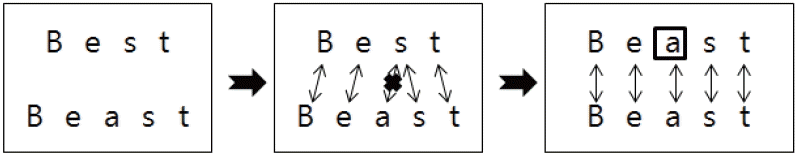##### Fig. 2
Example of a letter-based distance.##### Fig. 3
Example of a syllable-based distance.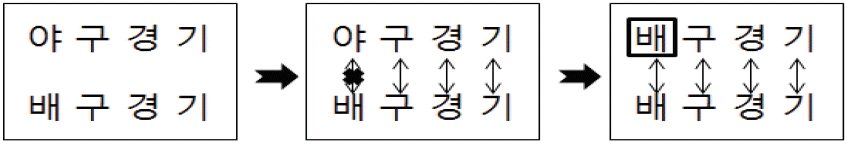##### Fig. 4
Example of a hybrid edit distance.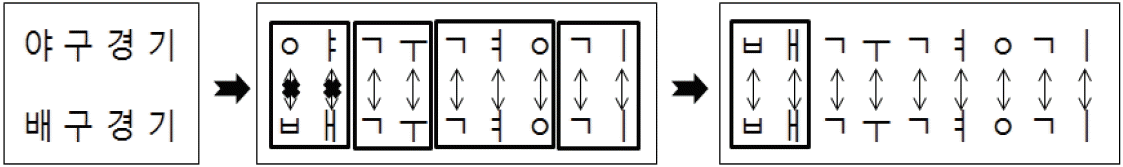##### Fig. 5
Example of conventional edit distance.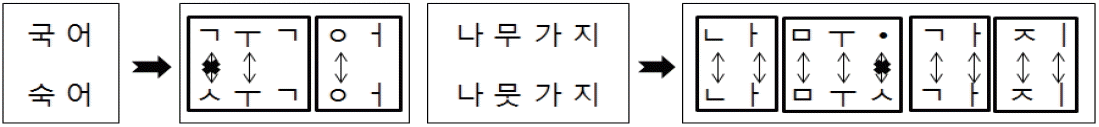##### Fig. 6
Normalization for similar word pairs.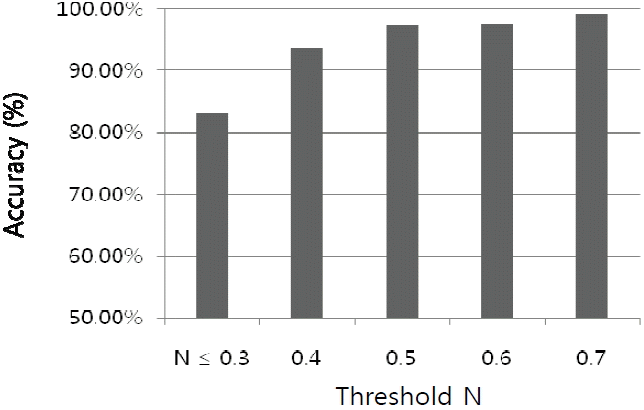##### Fig. 7
Normalization rate for non-similar word pairs.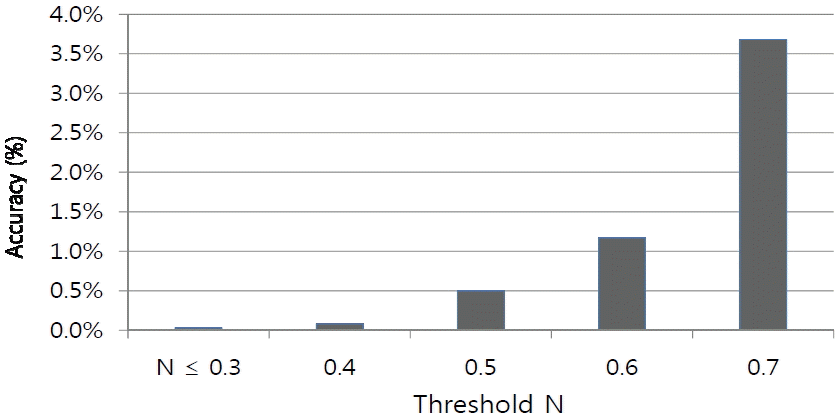##### Fig. 8
Accuracy of normalization factor.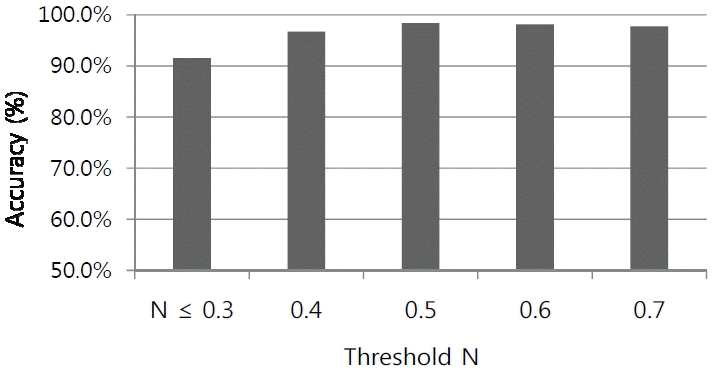##### Table 1
Phonetic representatives of consonants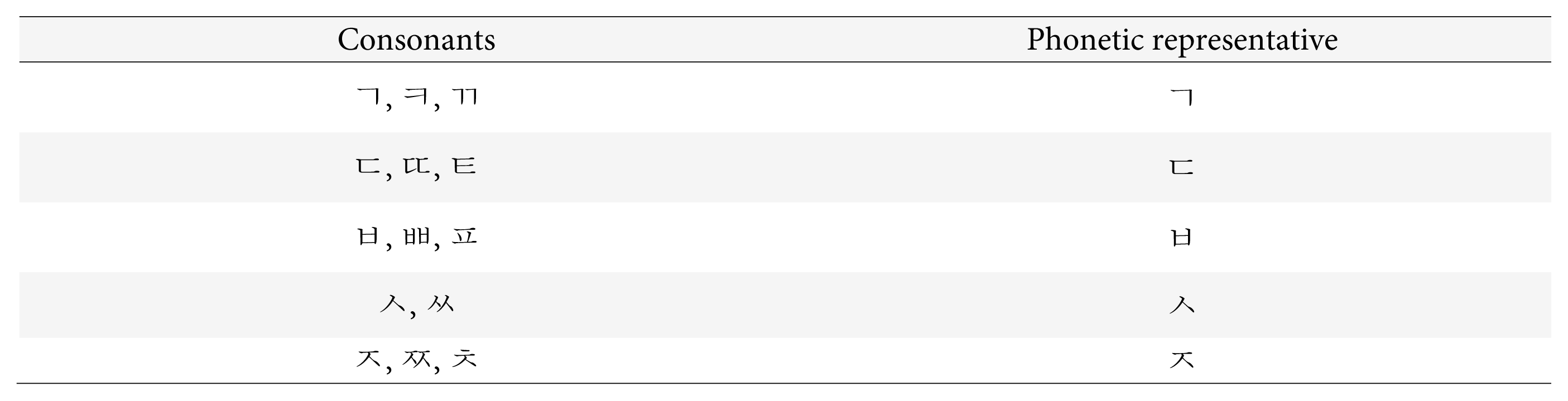##### Table 2
Edit distance for similar word pairs
Dist SylED LetED HybED PhoED NphoED
0 0 0 0 14.2 22.3
1 · 68.4 68.4 68.7 71.2
2 · 83.5 82.6 84.8 85.1
3 75.9 89.6 89.1 91.6 91.6
4 · 94.2 93.8 95.7 95.7
5 · 96.2 95.8 96.7 96.7
6 96.2 98.4 98.0 98.6 98.6
7 · 99.3 98.5 99.0 99.0
8 · 99.7 99.7 99.4 99.4
9 99.3 99.9 99.9 99.9 99.9
10 · 100.0 100.0 100.0 100.0
##### Table 3
Edit distance for non-similar word pairs
Dist SylED LetED HybED PhoED NphoED
0 0 0 0 0 0
1 · 1.9 1.9 2.3 2.4
2 · 13.5 11.6 12.1 12.5
3 31.6 42.7 35.0 32.4 33.1
4 · 56.1 50.0 50.6 54.4
5 · 100.0 76.8 75.6 75.6
6 79.1 100.0 93.4 91.6 91.9
7 · 100.0 100.0 100.0 100.0
8 · 100.0 100.0 100.0 100.0
9 99.3 100.0 100.0 100.0 100.0
10 · 100.0 100.0 100.0 100.0